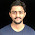Don't miss

## What is Flip-Flops|Explain S-R Flip-Flop

By on 02:06

Flip-Flop is a circuit which contains 2 outputs,one for normal value and other for opposite value or in simple words,it contains two states i.e. 0 and 1.

Type of Flip-Flop:-

1)S-R Flip-Flop

S-R Flip Flop:-

S-R stands for Set-Reset,in which reset means "clear".We can construct S-R flip flop with or without clock.In which '1'=Set and '0'=Reset.

Block Diagram of S-R flop flop:-

Truth Table:-

S R Q(n+1) Q(n+1)' Action
0 0 Q(n) Q(n)' No Change
0 1 01 Reset
1 0 10 Set
1 0 X X Forbidden

PS-NS Table(or Present State-Next State):-

Q(n) S R Q(n+1) Q(n+1)' Action
0 0 0l 0 1 No Change
0 0 1 0 1 Reset
0 1 0 1 0 Set
0 1 1 X X Forbidden
1 0 0 1 0 No change
1 0 1 0 1 Reset
1 1 0 1 0 Set
1 1 1 X X Forbidden

Excitation Table or Application Table:-

Q(n) Q(n+1) S R
0 0 0 X
0 1 1 0
1 0 0 1
1 1 X 0

Characteristic Equation of S-R flip flop:-

Q(n+1)=Q(n)'SR'+Q(n)R'

=R'(Q(n)S+Q(n))
=R'(Q(n)+s)
=Q(n)R'+SR'

Circuit Diagram of S-R flip flop using NAND gate:-

Circuit Diagram of S-R flip flop using NOR gate:-

If you have any query then leave your comments and don't forgot to follow me on Google+,Facebook,Twitter.

#### 1 comments:

1.nicely explained about SR Flip Flop